Navigation Panel:(These buttons explained below)Question Corner and Discussion Area

# What is i to the Power of i?

Asked by Oliver Varban, student, Earl Haig Secondary School on March 6, 1997:
I am interested in knowing what i to the power of i is.
The first question to address is what it means to raise one complex number to the power of another. There is a basic definition of what it means to raise e to a complex power, as described in the answer to an earlier question. Therefore, if z is any complex number for which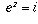,is a possible value for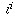.

What are the possible values for z? Well, if we write z = a + bi, then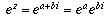. By de Moivre's theorem (explained in the answer to an earlier question),, so. This expression equals i exactly when a=0, cos(b)=0, and sin(b)=1. This occurs when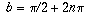for some integer n, so the possible values of z are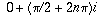.

Therefore, the values ofarefor any integer n.

Note that there is more than one value for, just as 2 and -2 are both square roots of 4. (However, while the square roots of a number always have the same magnitude even if they differ in sign, the values ofhave different magnitudes). The principal value ofwould be--the case where n=0.

It's also interesting to note that all these values ofare real numbers.

[ Submit Your Own Question ] [ Create a Discussion Topic ]

This part of the site maintained by (No Current Maintainers)
Last updated: April 19, 1999
Original Web Site Creator / Mathematical Content Developer: Philip Spencer
Current Network Coordinator and Contact Person: Joel Chan - mathnet@math.toronto.edu

Navigation Panel: